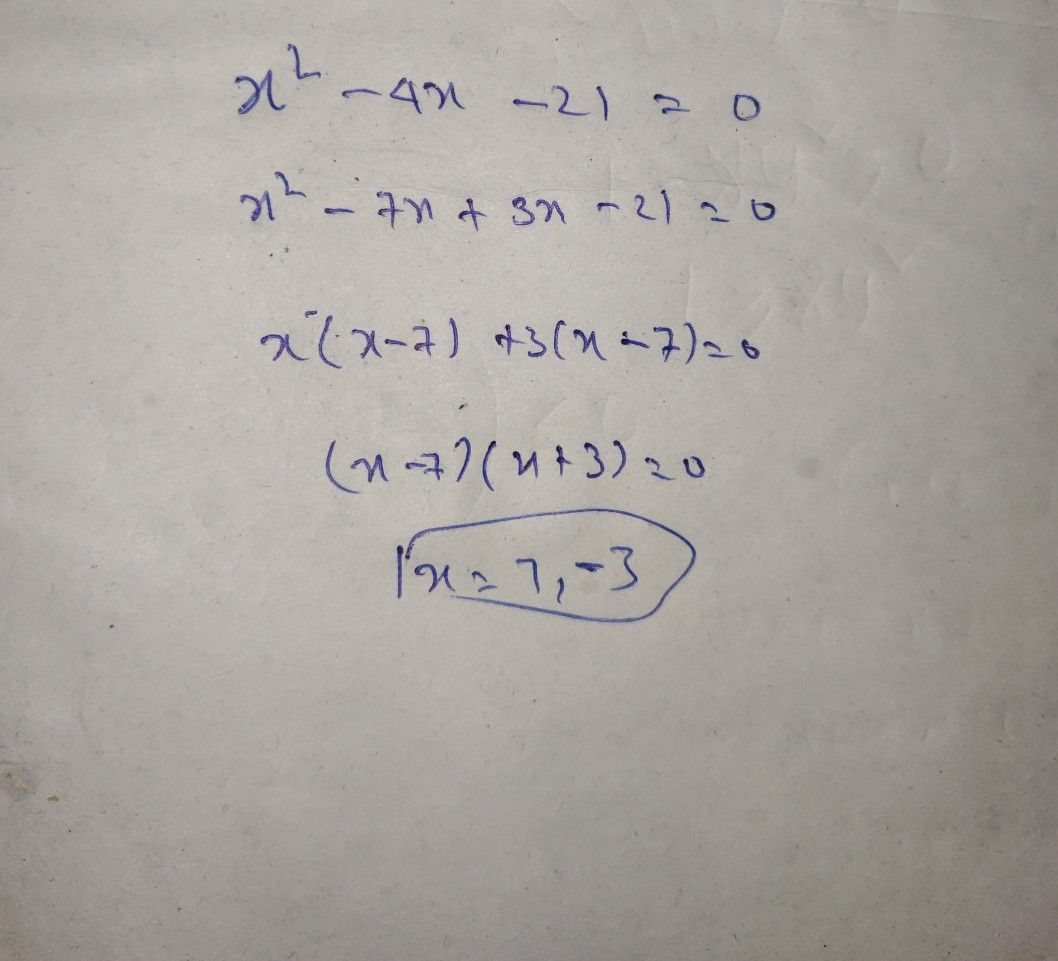Symbol
Problem$2\right)=0$ $\dfrac {x^{2}-\right)C-} {x^{2}-Mx-}a1=0$ $s\right)x^{2}-9$ $QS$ $-18Q=O$
10th-13th grade
Other
SolutionQanda teacher - RUCHIPlz evaluate my answer and rate my session 5 star
If you any doubt ask me dear
Like meStudent
not correct
mam
hlo